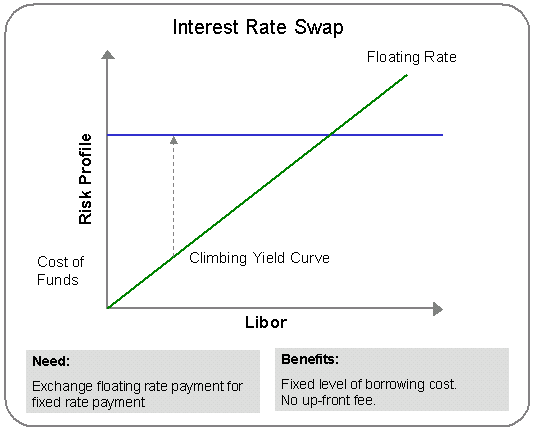# How Interest Rate Swaps Work?

An interest rate swap is an exchange of cash flows between two parties where party A pays a fixed rate and receives a floating rate and party B receives a fixed rate and pays the floating rate. In essence, party A and party B, known as counterparties, agree to exchange a series of cash flows in the future for a specified period of time. Since only cash follows are exchanged, principal payments are not involved.

#### Example 1

Assume a \$100 million, three year paying fixed interest rate swap is set at 5.50% versus 6 month LIBOR (assumed at 3.50%). This transaction hedges the Treasurer against higher rates and costs nothing to enter into. However, exchanging of cash flows negatively impacts the company in the beginning as they are paying 5.50% to the counterparty and in return receiving 3.50% on the initial rate setting. Consequently, the steepness of the yield curve makes hedging with swaps very difficult for Treasurers.

The following diagram depicts an interest rate swap.#### Example 2

In another example, if a manufacturing firm wanted to “swap out” its floating rate debt, its all-in-cost of fixed rate debt would be quoted at 7.50% assuming the following information.

 Term of floating debt 5 years 5-year Treasury yield 5.70% Loan spread 150 basis points 3-month Libor 3.50% Swap spread 30 days

All in cost approximately = 5.70 + 0.30 + 1.50 = 7.50%

The manufacturing firm will now pay 7.50% over the next five years and receive a floating 3-month Libor over the same term.